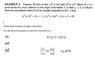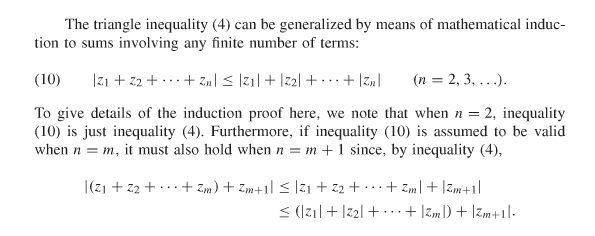# Complex Numbers Moduli Problem

• I
• SamitC

#### SamitC

This may be a simple thing but due to some reason I am not able to understand.
I am not able to understand an example from Brown-Churchill book. I have provided the screenshot in the attached picture. Request help.

#### Attachments

•InEq.jpg
25.1 KB · Views: 455

I think you may have copied the wrong equation for (9). The triangle inequality says that ##|a+b|\leq|a|+|b|##. Applying that to your equation we get:

$$|z^3+3z^2-2z+1|\leq|z^3+3z^2-2z|+|1|\leq|z^3+3z^2|+|-2z|+|1|\leq|z^3|+|3z^2|+|-2z|+|1|$$
[applying the triangle inequality three times in succession]
$$=|z|^3+3|z|^2+|-2||z|+1$$
[applying (8) ]
$$=|z|^3+3|z|^2+2|z|+1<2^3+3\cdot 2^2+2\cdot 2+1=25$$

EDIT: Just saw Samy's post. I don't have the book but, based on that picture, it looks more likely a typo in that the ref to (9) should be to (10), rather than you miscopying it.

•SamitC
May well be a typo. He is referring to the following:•SamitC
I think you may have copied the wrong equation for (9). The triangle inequality says that ##|a+b|\leq|a|+|b|##. Applying that to your equation we get:

$$|z^3+3z^2-2z+1|\leq|z^3+3z^2-2z|+|1|\leq|z^3+3z^2|+|-2z|+|1|\leq|z^3|+|3z^2|+|-2z|+|1|$$
[applying the triangle inequality three times in succession]
$$=|z|^3+3|z|^2+|-2||z|+1$$
[applying (8) ]
$$=|z|^3+3|z|^2+2|z|+1<2^3+3\cdot 2^2+2\cdot 2+1=25$$

EDIT: Just saw Samy's post. I don't have the book but, based on that picture, it looks more likely a typo in that the ref to (9) should be to (10), rather than you miscopying it.
Thank you very much.